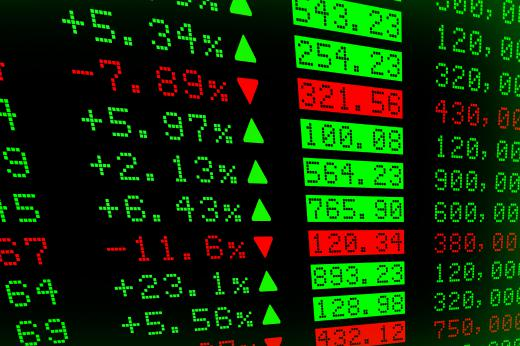Finance
Fact Checked

# What is a Risk Adjusted Return?

Dana DeCecco
Dana DeCecco

A risk adjusted return is the numerical value of a risk assessment calculation applied to an investment. The return produced by an investment is compared to the risk of the investment, resulting in a ratio. The risk adjusted return can be applied to individual securities, a portfolio of securities, or a fund. Different forms of risk measurement provide investors with diverse methods of evaluating the risk adjusted return.

Analyzing the performance of investments is typically based on the return on investment (ROI). The ROI is a simple performance ratio used to evaluate the efficiency of an investment. The quick and easy ROI method does not take into account the amount of risk assumed to create the profit. Risk is the most important consideration when trading in financial markets.Riskier investments tend to produce larger profits or losses, while investments with less risk produce smaller profits.

Riskier investments tend to produce larger profits or losses, while investments with less risk produce smaller profits. Investments with a theoretical risk of zero, such as US treasury bills, produce the smallest returns. Comparing investments using a risk adjusted return method helps the investor decide which investment is more suited to purpose and risk tolerance.

Risk adjusted return on capital (RAROC) is a basic formula that takes into account the variable of risk. It provides a useful way to compare diverse investments with different risk levels. The rational investor comparing two investments with the same return would select the one with the lowest risk. For the investor to assume more risk, the investment would need to produce a relatively greater risk-to-reward ratio.

The Sharpe ratio, developed by William F. Sharpe, indicates whether the performance of an investment is the result of assuming excess risk. Various market conditions, such as a strong bull market, can produce strong profits. The same investment under different market conditions may produce different results. The question is whether the investment produces a large profit because of random events or wise investment decisions.

Variations of the Sharpe ratio employ different volatility variables to refine analysis of market conditions. Such formulas include the Treynor ratio, the Sortino ratio, and the modified Sharpe ratio. These variations use statistical measurements such as standard deviation, r-squared, and beta. Comparisons to the risk-free rate and benchmark returns are used to determine the quality of the investment.

All risk adjusted return methods are widely used but still theoretical in nature. If the perfect formula were developed, there would be no need for discretion, and all investments would be black or white. These analysis methods are best used when comparing an investment to relevant market benchmarks. Perhaps the next formula will provide a ratio comparing ratios.

## You might also Like